The NPAR1WAY Procedure

Hodges-Lehmann Estimation of Location Shift

If you specify the HL option, PROC NPAR1WAY computes the Hodges-Lehmann estimate of location shift for two-sample data. PROC NPAR1WAY also provides confidence limits for the location shift. These confidence limits are sometimes called Moses confidence limits. You can set the level of the confidence limits with the ALPHA= option. The default is ALPHA=0.05, which produces 95% confidence limits. Additionally, you can request exact confidence limits for the location shift by specifying the HL option in the EXACT statement.

The Hodges-Lehmann estimator of location shift is associated with the Wilcoxon linear rank statistic. See Hollander and Wolfe (1999) and Hodges and Lehmann (1983) for details.

PROC NPAR1WAY computes the Hodges-Lehmann estimate
as the median of all paired differences between observations in the two samples (classes), which can be written as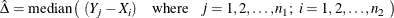The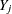are observations in class 1, the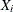are observations in class 2, and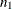and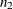denote the number of observations in class 1 and class 2, respectively.

By default, PROC NPAR1WAY uses the larger of the two classes as the reference class X (class 2). If both class have the same number of observations, PROC NPAR1WAY uses the class that appears second in the input data set as the reference class. You can specify the reference class by using the HL(REFCLASS=) option. REFCLASS=1 refers to the first class that is listed in the Wilcoxon Scores table, and REFCLASS=2 refers to the second class in the table. REFCLASS=class-value identifies the reference class by the formatted value of the CLASS variable.

Let m denote the total number of differences (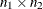), and let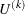denote the kth value of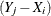among the ordered differences. When m is an odd number, then the median difference is the value with rank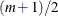,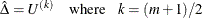When m is an even number, the median difference is the average of the values with ranks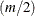and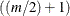,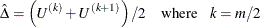Following Hollander and Wolfe (1999), the asymptotic lower and upper confidence limits for the location shift are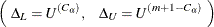where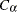is the largest integer less than or equal to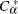, which is computed as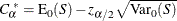where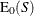and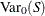are the expected value and variance, respectively, of the Wilcoxon statistic S under the null hypothesis (as described in the section Simple Linear Rank Tests for Two-Sample Data), and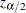is the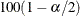percentile of the standard normal distribution. For Wilcoxon rank scores,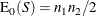When there are no tied values,for Wilcoxon scores equalsPROC NPAR1WAY displays the midpoint of the confidence interval, which can also be used as an estimate of location shift. See Lehmann (1963) for details. Additionally, PROC NPAR1WAY provides an estimate of the asymptotic standard error ofbased on the length of the confidence interval, which is computed as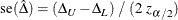Exact Confidence Limits

If you specify the HL option in the EXACT statement, PROC NPAR1WAY computes exact confidence limits for the location shift between the two samples. As for the asymptotic confidence limits, you can set the confidence level with the ALPHA= option. The default is ALPHA=0.05, which produces 95% confidence limits.

PROC NPAR1WAY computes exact confidence limits for the location shift as described in Randles and Wolfe (1979, p. 180). PROC NPAR1WAY first generates the exact conditional distribution of the Mann-Whitney U statistic, which equals the number of pairwise differences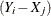that are positive, plus half the number of pairwise differences that are zero. The Mann-Whitney statistic is defined as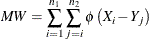where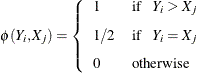From the exact conditional distribution of the Mann-Whitney statistic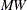, PROC NPAR1WAY chooses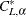as the largest value such that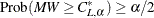. Roundingup to the nearest integer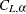, the lower confidence limit equals the differencethat has a rank of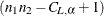.

To find the upper confidence limit, PROC NPAR1WAY chooses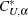as the smallest value such that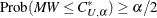. Roundingdown to the nearest integer, the upper confidence limit equals the differencethat has a rank of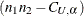.

Because this is a discrete problem, the confidence coefficient for these exact confidence limits is not exactly (1 –) but is at least (1 –). Thus, these confidence limits are conservative.